# What is the difference between join and merge in Pandas?

• Last Updated : 24 Jan, 2021

Pandas provide various facilities for easily combining Series or DataFrame with various kinds of set logic for the indexes and relational algebra functionality in the case of join / merge-type operations.

Both join and merge can be used to combines two dataframes but the join method combines two dataframes on the basis of their indexes whereas the merge method is more versatile and allows us to specify columns beside the index to join on for both dataframes.

Attention geek! Strengthen your foundations with the Python Programming Foundation Course and learn the basics.

To begin with, your interview preparations Enhance your Data Structures concepts with the Python DS Course. And to begin with your Machine Learning Journey, join the Machine Learning - Basic Level Course

Let’s first create two dataframes to show the effect of the two methods.

## Python3

 `import` `pandas as pd`` ` `# Creating the two dataframes``left ``=` `pd.DataFrame([[``'a'``, ``1``], [``'b'``, ``2``]], ``list``(``'XY'``), ``list``(``'PQ'``))``right ``=` `pd.DataFrame([[``'c'``, ``3``], [``'d'``, ``4``]], ``list``(``'XY'``), ``list``(``'PR'``))`

Output: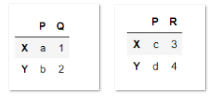Now let’s see the effect of the two methods on the dataframes one by one.

## join

The join method takes two dataframes and joins them on their indexes (technically, you can pick the column to join on for the left dataframe). If there are overlapping columns, the join will want you to add a suffix to the overlapping column name from the left dataframe. Our two dataframes do have an overlapping column name P.

Example :

## Python3

 `joined_df ``=` `left.join(right, lsuffix``=``'_'``)``print``(joined_df)`

Output :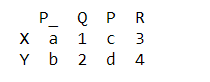Notice the index is preserved and we have four columns.nWe can also separately specify a specific column of the left dataframe with a parameter on to use as the join key, but it will still use the index from the right.

Example :

## Python3

 `joined_df2 ``=` `left.reset_index().join(right, on``=``'index'``, lsuffix``=``'_'``)``print``(joined_df2)`

Output :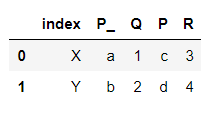## merge

At a basic level, merge more or less does the same thing as join. Both methods are used to combine two dataframes together, but merge is more versatile, it requires specifying the columns as a merge key. We can specify the overlapping columns with parameter on, or can separately specify it with left_on and right_on parameters.

Example :

## Python3

 `merged_df ``=` `left.merge(right, on``=``'P'``, how``=``'outer'``)``print``(merged_df)`

Output :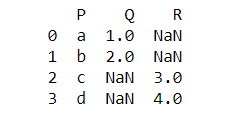Here, notice that the merge method destroyed the index.

We can explicitly specify that we are merging on the basis of index with the left_index or right_index parameter.

Example :

## Python3

 `merged_df ``=` `left.merge(right, left_index``=``True``,``                       ``right_index``=``True``, suffixes``=``[``'_'``, ''])``print``(merged_df)`

Output :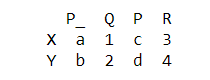My Personal Notes arrow_drop_up